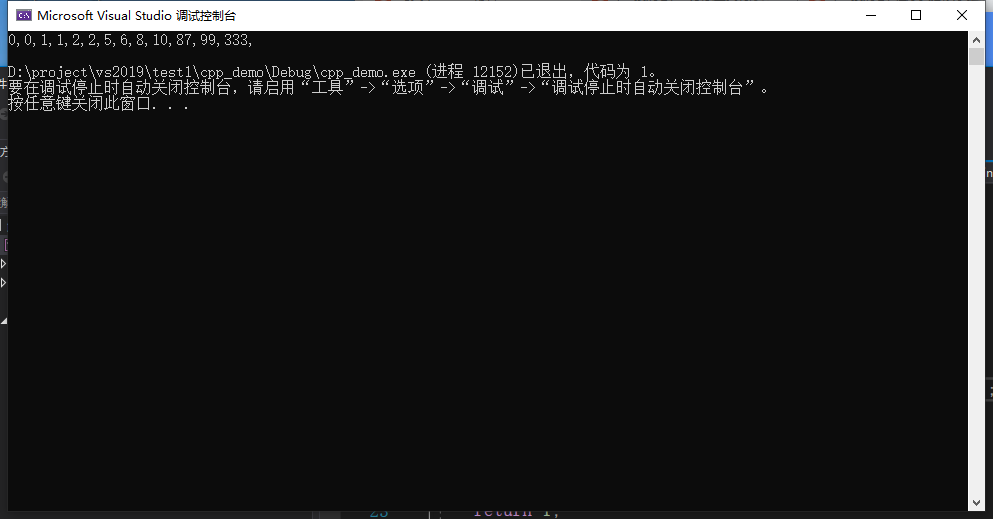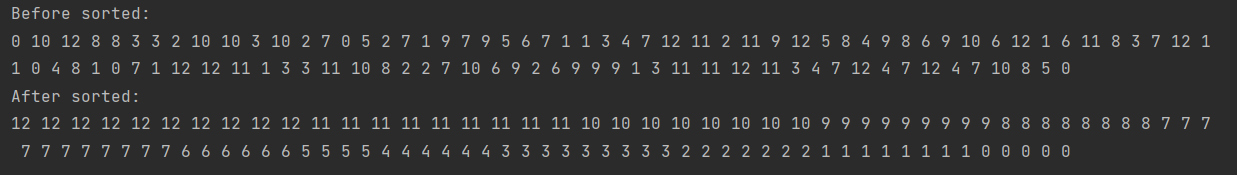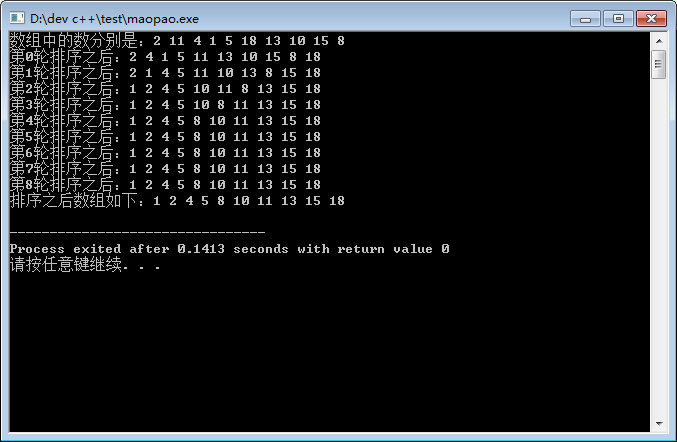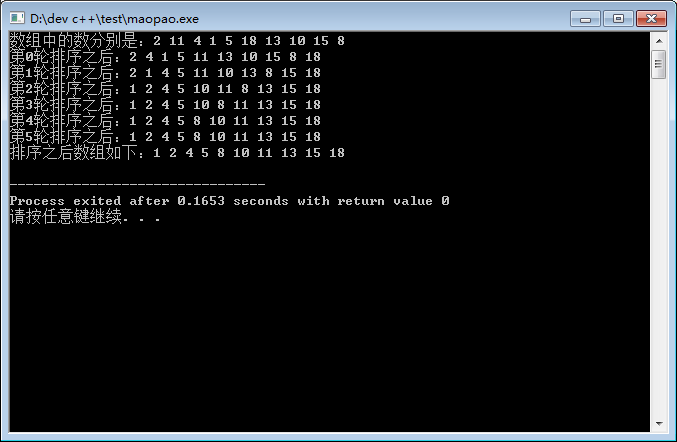• ，程序实现冒泡排序十万个数（调用），可以改成输入。并附加程序运行计时，用于测试时间复杂度，可以移除
• 冒泡排序算法比较简单，就是从左到右两两比较大小，比如升序排序，则把较大元素放到后面，第一轮结束后，开始第二轮比较排序；第二轮到第n-1个元素，一只到第n-1轮到比较最开始的两个元素则终止比较， 下面实现代码...
冒泡排序算法比较简单，算法思想主要是双层循环，外层循环进行n-1趟排序，内层循环依次从左到右紧挨着的两个元素比较大小，内层循环的终止条件由外层循环的变量j控制。
注意：冒泡排序时间复杂度是O(n^2)，空间复杂度为O(1)，是稳定排序算法（排序前后相对位置不变）
下面实现代码：
#include <iostream>
#include <vector>

using namespace std;
class Sort {
public:
void bubble(vector<int>& nums) {
for (int j = nums.size() - 1; j > 0; --j) // 外层循环控制内层循环终止条件
for (int i = 0; i < j; ++i) // 内层循环每趟比较出一个最大值放“最后面”
if (nums[i] >= nums[i + 1]) // 前面比后面大则交换
std::swap(nums[i], nums[i + 1]);
}
};

int main()
{
vector<int> nums = { 2,0,1,6,8,10,5,99,87,333,2,0,1 };
Sort{}.bubble(nums);
for (const auto& it : nums)
cout << it << ",";
cout << endl;
return 1;
}展开全文• C++ 冒泡排序算法实现代码，用C++编写的冒泡排序算法代码。
• 冒泡排序 两两比较相邻记录的关键字，如果不符合顺序要求则交换，直到没有不符合顺序要求的为止。 排序示意图 代码实现 源代码： #include <iostream> using namespace std; void bublesort(int *arr,int len)...
冒泡排序
两两比较相邻记录的关键字，如果不符合顺序要求则交换，直到没有不符合顺序要求的为止。
算法过程
第1个元素开始，比较相邻的两个元素，如果前者比后者大，则交换，一直到最后一个元素（最后一个元素为最大，类似冒泡泡的过程）然后从第2个元素重复以上过程，直到所有的元素均被遍历到
算法评价
需要不断地交换元素，时间复杂度较高，为

O

(

n

2

)

O(n^2)

是一种稳定的排序算法
代码实现
源代码：
#include <iostream>
using namespace std;
void bublesort(int *arr,int len){
int i,j,tmp;
for(i=0;i<len;i++){
for(j=0;j<len-1;j++){
if(arr[j]>arr[j+1]){//相邻比较，如果满足条件则交换
tmp=arr[j];
arr[j]=arr[j+1];
arr[j+1]=tmp;
}
}
}
}
int main()
{
int arr[]={9,1,5,8,3,7,4,6,2};
cout<<"排序前顺序："<<endl;
for(int i=0;i<9;i++)cout<<arr[i];
bublesort(arr,9);
cout<<"\n排序后顺序："<<endl;
for(int i=0;i<9;i++)cout<<arr[i];
return 0;
}

运行结果：
排序前顺序：
915837462
排序后顺序：
123456789

展开全文算法
• 冒泡排序：通过不断比较数组中两个数的位置，按照一定顺序（升序或者降序）将每个数字一点一点排好. 举例说明 需要排序数组： 1 2 3 4 5 要求将其从大到小重新排序，则步骤为： Round 1：从第一位数字开始进行...
原理
算法简介
冒泡排序：通过不断比较数组中两个数的位置，按照一定顺序（升序或者降序）将每个数字一点一点排好.
举例说明
需要排序数组：
12345
要求将其从大到小重新排序，则步骤为：
Round 1：从第一位数字开始进行循环比较，把小的数字换到后一位去
comparision ①
21345
comparision ②
23145
comparision ③
23415
comparision ④
23451
Round 2：再次从第一位数字开始进行循环比较
comparision ①
32451
comparision ②
34251
comparision ③
34521
因为最后1位数字已经肯定是最小的那1位了，所以无需比对最后1位
Round 3：再次从第一位数字开始进行循环比较
comparision ①
43521
comparision ②
45321
因为最后2位数字已经肯定是最小的那2位了，所以无需比对最后2位
Round 4：再次从第一位数字开始进行循环比较
comparision ①
54321
因为最后3位数字已经肯定是最小的那2位了，所以无需比对最后3位
好了，现在排序已经完成，我们总结一下 round 和 comparision 比对次数的关系：
round indexcomparision(s)sum145235325415
数组长度记为N，在本例中，N = 5，由此我们可以得到结论：
1）round 只要运行 N - 1 次就行
2）comparision 每次比对的总次数为 N - round index
因此，冒泡排序算法可以使用2层循环来实现，第一层循环表示round（即round index），第二层循环表示cIndex（即compare Index）.

冒泡排序代码
代码如下（整数数组，从大到小排列）：
void bubbleSort(int arr[], int len) {
int round = 0;
int cIndex = 0; // short for compareIndex
int temp = 0;

for (round = 1; round < len; round++) {
for (cIndex = 0; cIndex < len - round; cIndex++) {
if (arr[cIndex + 1] > arr[cIndex]) {
temp = arr[cIndex];
arr[cIndex] = arr[cIndex + 1];
arr[cIndex + 1] = temp;
}
}
}
}

随手改了个浮点数版本的也放上来：
// 浮点数改进版
void dBubbleSort(double arr[], int len) {
int round = 0;
int cIndex = 0;
double temp = 0.0;

for (round = 1; round < len; round++) {
for (cIndex = 0; cIndex < len - round; cIndex++) {
if (arr[cIndex + 1] > arr[cIndex]) {
temp = arr[cIndex];
arr[cIndex] = arr[cIndex + 1];
arr[cIndex + 1] = temp;
}
}
}
}

时间复杂度
假设需要排序的数组长度为n，则：
该算法中循环语句执行总次数：.
时间复杂度取 T(n) 最高次项，即 .

完整可运行代码：产生随机数 + 排序 + 打印检验

** C++产生随机数，可参考我之前的博客：C++产生随机数的几种方法

以整数版本代码为例：
#include <iostream>
#include <random>
#include <ctime>

// 产生整数随机数
void iRand(int arr[], int len, int min, int max) {
std::default_random_engine e;
e.seed(time(0));
std::uniform_int_distribution<int> u(min,max);

int i = 0;
for (i = 0; i < len; i++) arr[i] = u(e);
}

// 整数数组排序
void bubbleSort(int arr[], int len) {
int round = 0;
int cIndex = 0; // short for compareIndex
int temp = 0;

for (round = 1; round < len; round++) {
for (cIndex = 0; cIndex < len - round; cIndex++) {
if (arr[cIndex + 1] > arr[cIndex]) {
temp = arr[cIndex];
arr[cIndex] = arr[cIndex + 1];
arr[cIndex + 1] = temp;
}
}
}
}

// 设置参数
const int LEN = 100;
const int I_MIN = 0;
const int I_MAX = 12;

int main() {
int arr[LEN] = {0};

// 产生实数随机数组
iRand(arr, LEN, I_MIN, I_MAX);
// 打印原始数组
std::cout << "Before sorted:" << std::endl;
int i = 0;
for (i = 0; i < LEN; i++) {
std::cout << arr[i] << " ";
}
std::cout << " " << std::endl;

// 冒泡排序
bubbleSort(arr, LEN);
// 打印排序后数组
std::cout << "After sorted:" << std::endl;
for (i = 0; i < LEN; i++) {
std::cout << arr[i] << " ";
}
std::cout << " " << std::endl;

return 0;
}
结果：展开全文算法
• c++冒泡排序算法实现 冒泡排序，是一种计算机科学领域的较简单的排序算法。它通过遍历要排序的元素，依次比较相邻元素的大小，如果前面的元素大于后面的元素，则交换两个元素的位置。因此，在第一趟遍历完成时，会把...
c++冒泡排序算法实现
冒泡排序，是一种计算机科学领域的较简单的排序算法。它通过遍历要排序的元素，依次比较相邻元素的大小，如果前面的元素大于后面的元素，则交换两个元素的位置。因此，在第一趟遍历完成时，会把最大的元素排在最末尾，第二趟遍历完成时，会把次大的元素排在倒数第二位，依次类推直到要排序的元素以升序的方式排列。由于该过程类似于鱼吐的泡泡一样向上浮，所以冒泡排序也由此得名。 接下来来看一下c++如何实现冒泡排序的。
#include <iostream>
using namespace std;
int main()
{
int a = {2,11,4,1,5,18,13,10,15,8};
cout << "数组中的数分别是：";
for(int i = 0;i<10;i++)
cout << a[i] << " ";
cout << endl;
for(int i = 0;i<9;i++)
{
for(int j = 0;j<9-i;j++)
{
int temp = 0;
if(a[j]>a[j+1])//如果前面的元素大于后面的元素，则交换位置
{
temp = a[j];
a[j] = a[j+1];
a[j+1] = temp;
}
}
cout << "第" << i << "轮排序之后：";
for(int k =0;k<10;k++)
{
cout << a[k] << " ";
}
cout << endl;
}
cout << "排序之后数组如下：";
for(int i = 0;i<10;i++)
cout << a[i] << " ";
cout << endl;
return 0;
}

运行代码，结果如下：从排序的结果可以看出，从最大数，次大数…等会依次被放置在正确的位置上。当然再仔细观察一下，可以看出在第4（从0开始算）轮排序之后，所有的数据都已经被排好序了，所以第4轮之后的所有排序都是无用功。因此这里可以想一下，是否可以判断数据有序时，提前结束排序呢？这种判断的依据是什么呢？ 很显然，当某一轮遍历完成时，没有进行交换数据位置的操作，就可以说明数据是有序的。因此可以增加一个标志位，来记录是否有进行数据交换。改进的冒泡排序具体实现如下：
#include <iostream>
using namespace std;
int main()
{
bool flag = false;
int a = {2,11,4,1,5,18,13,10,15,8};
cout << "数组中的数分别是：";
for(int i = 0;i<10;i++)
cout << a[i] << " ";
cout << endl;
for(int i = 0;i<9&&!flag;i++)
{
flag = true;
for(int j = 0;j<9-i;j++)
{
int temp = 0;
if(a[j]>a[j+1])
{
temp = a[j];
a[j] = a[j+1];
a[j+1] = temp;
flag = false;
}
}
cout << "第" << i << "轮排序之后：";
for(int k =0;k<10;k++)
{
cout << a[k] << " ";
}
cout << endl;
}
cout << "排序之后数组如下：";
for(int i = 0;i<10;i++)
cout << a[i] << " ";
cout << endl;
return 0;
}

	运行代码，结果如下：在第四轮排序之后可以看到数据已经有序了，再进行第五轮遍历时，没有进行交换数据的操作，所以设定的flag = true，此时再进行i<9&&!flag的判断时，条件不成立，可以提前结束排序，以免再有多余的遍历和比较的过程。 感兴趣的朋友们可以关注我，一起学习C++吧！
展开全文• 冒泡排序是非常经典的排序算法 比如有8个随机的数字，现在把他们升序排列（有小到大排列出来）。当然想到的就是两个比较。这样会有好多轮比较。 第一轮：从左往右开始，左边的和右边的比较，左边 > 右边，交换...
• 冒泡排序的原理： 1.比较相邻的两个元素（例如：升序排列），如果第一个元素大于第二个元素，则交换元素的位置，反之不变。 2.对每一对相邻的两个元素，重复步骤一；从开始第一对到最后一对。 3.针对所有的元素...
• * * * * * * * * * 冒泡排序算法 株洲市第二中学 信息技术组 刘辉琴 杜新宇 将下面一组无序的数据从小到大排列 { 49,38,65,97,76,13,27,49 } 数据如何存储 活动 一维数组 定义一维数组 int r 数组元素为 r, r...
• 主要为大家详细介绍了C++实现双向冒泡排序算法，文中示例代码介绍的非常详细，具有一定的参考价值，感兴趣的小伙伴们可以参考一下
•  冒泡排序算法的运作如下：（从后往前）  1.比较相邻的元素。如果第一个比第二个大，就交换他们两个。  2.对每一对相邻元素作同样的工作，从开始第一对到结尾的最后一对。在这一点，最后的元素应该会是最大的数...
• 首先说一下冒泡排序的基本算法思想： 它重复地走访过要排序的元素列，依次比较两个相邻的元素，如果顺序（如从大到小、首字母从Z到A）错误就把他们交换过来。 这个算法的名字由来是因为越小的元素会经由交换慢慢...数据结构
• 冒泡排序c++实现冒泡排序代码 冒泡排序的基本思想： 冒泡排序的核心是对所有相邻数组元素的值进行比较，如果是逆序(a[i]>a[i+1])，则两者交换，最终达到有序。 步骤： （1）比较第一个数a和第二个数a,...
• 实现对一串数字的冒泡排序处理 for(i = 1; i ; i++) { flag = 0; for(j = 0; j ; j++) { if(a[j].key > a[j+1].key) { flag = 1; temp = a[j]; a[j] = a[j+1]; a[j+1] = temp; } ...
• 冒泡排序算法是一种简单的排序算法，该算法的思想就是将元素进行两两互相交换的过程，比如有一个10个元素的数组，用冒泡排序的步骤有： 1、先判断第0个元素与第一个元素之间的关系，如果第0个元素比第一个元素小，...
• ## 冒泡排序法（C++实现）

万次阅读 多人点赞 2018-04-07 16:09:02
用户从键盘输入N，然后输入N个实数，使用冒泡排序方法对这N个元素排序，输出排序后的数据。 何谓冒泡排序法 (1)两两比较相邻元素A(I)和A(I+1)(I=1,2,…N-1),如果A(I)>A(I+1),则交换A(I)和A(I+1)的位置； (2)对剩下的...
• //计数排序 TypeName void Sort(T R[],int n) { int l = 0, r = n - 1; while (l) { for (int i = l; i ; i++)//由左向右扫描，将最大值置于右边 { if (R[i]>R[i + 1]) SWAP(R, i, i + 1); } --r;...
• 冒泡排序（Bubble Sort），是一种计算机科学领域的较简单的排序算法。 它重复地走访过要排序的数列，一次比较两个元素，如果他们的顺序错误就把他们交换过来。走访数列的工作是重复地进行直到没有再需要交换，也...
• ## C++简单排序算法之冒泡排序

万次阅读 多人点赞 2016-09-08 22:01:13
冒泡排序（Bubble Sort），是一种...冒泡排序算法的运作如下：（从后往前）比较相邻的元素。如果第一个比第二个大，就交换他们两个。 对每一对相邻元素作同样的工作，从开始第一对到结尾的最后一对。在这一点，最后
• 普通排序 普通排序的效率较低，工作原理就是将从下到上一一比较，每一轮都将没排序的数据最小的或最大的排在最上方 也就是说，如果要对n分个数排序，就要做（n-1）轮，做（1+2+3+……+(n-1)）次，运行速度较慢 #...快速排序 算法
• 冒泡排序的思想是：从头访问待排序的数列，每次比较相邻的两个元素，将他们的顺序按照从大到小或者从小到大调整过来，直到完成整个数列所有元素的顺序调整。 动态效果示意图如下： C++代码 #include <iostream&...
• 冒泡排序是一种非常简单的排序算法，它重复地走访过要排序的数列，每一次比较两个数，按照升序或降序的规则，对比较的两个数进行交换。比如现在我要对以下数据进行排序： 10 3 8 0 6 9 2 当使用冒泡排序进行升序排序...
• C++排序算法冒泡排序_代码 template< class T> void swaps(T& a, T& b) { T temp = a; a = b; b = temp; return; } class Myset { private: int* arr; int size; public: Myset() { arr = ...数据结构
• 冒泡排序算法为最简单的排序算法，易于实现，也容易理解，下面为实现代码： #include <iostream> using namespace std; void sort(int *arr,int length) //传入数组首地址和数组长度 { int temp; for ...
• 冒泡排序算法C++函数模板，适用于多种数据类型，如int,float,double等...

# 冒泡排序算法c++c++ 订阅